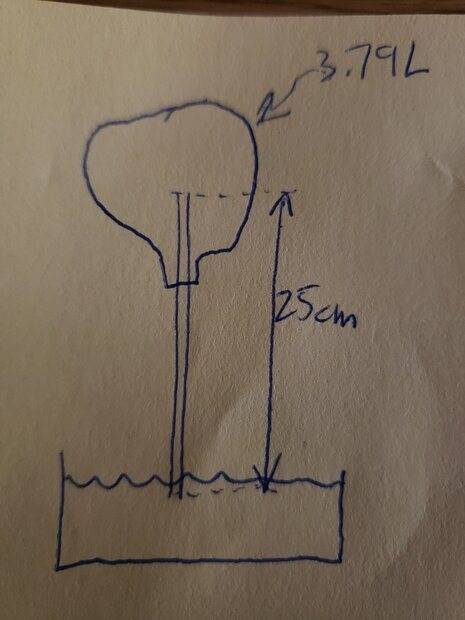# Volume of water consumed by vacuum

• I
northmop
I'm trying to do the math for a backyard thermodynamic pump experiment, and getting stuck. Suppose an empty 3.79 liter container was airlocked by a vertical 25 cm long empty tube whose end sits at the top of an unlimited supply of water in a basin. The tube protrudes through the container such that there is a length of tube above the base of the container so that any water entering the container cannot escape back down the tube and into the basin. If the temperature of the external environment, water, and gas are all constant (295°K), what volume of water (density of 1000 kg/m3) would enter the container if the pressure differential between of the external environment (100 kPa) and the inside the container (96 kPa) is 4 kPa?

I know that some of the vacuum pressure will be consumed to maintain the 25 cm height of the water column in the tube. This means that 4 kPa - (9.80665 m/s2 * 1000 kg/m3 * 0.25 m) = 1.54834 kPa is "available" to vacuum up the water from the basin into the container. What I'm getting stuck with is how to calculate the hypothetical volume of water that will get sucked up and stored in the container: my head is mush after thinking about this too long. Would you please be so kind as to share the formulas used to figure this out?

Last edited:

northmop
I wanted to post this separately so that I don't confuse the question. Here's the short of my experiment. During the day the container warms up. As night passes, the container cools down and sucks up liquid. Using the Ideal Gas Law, one can determine a change in volume from the hottest point in the day (H) and the coolest point in the day (C). If the container was malleable, the volume of the container would change, either by expanding or collapsing, until the internal pressure of the container was equalized with the pressure of its external environment. That change in volume, ΔV, can be calculated as:

ΔV = VH – VC
VC = VH – ΔV
PH VH = n R TH
n = ( PH VH ) / ( R TH )
PC VC = n R TC
PC ( VH – ΔV ) = [ ( PH VH ) / ( R TH ) ] ( R TC )
PC VH – PC ΔV = ( PH VH R TC ) / ( R TH )
PC VH – PC ΔV = ( PH VH TC ) / ( TH )
– PC ΔV = ( PH VH TC ) / ( TH ) – ( PC VH )
PC ΔV = – ( PH VH TC ) / ( TH ) + ( PC VH )
ΔV = [ – ( PH VH TC ) / ( TH ) + ( PC VH ) ] / PC
ΔV = – ( PH VH TC ) / ( PC TH ) + ( PC VH / PC )
ΔV = – ( PH VH TC ) / ( PC TH ) + VH
ΔV = VH – ( PH VH TC ) / ( PC TH )
ΔV = VH [ 1 – ( PH TC ) / ( PC TH ) ]
ΔV = VH [ 1 – ( PH / PC ) ( TC / TH ) ]​

However, the jug is not malleable meaning that VH=VC and V is therefore a constant. A volume of matter will enter the jug when ΔV > 0 and exist the jug when ΔV < 0 in order to equalize its pressure with that of the external environment, PE. As my concern is when ΔV > 0, PC = PE and I get a final equation of:

ΔV = V [ 1 – ( PH / PE ) ( TC / TH ) ]​

What gives me the willies is that, though this theoretically will yield the volume of water sucked into the straw and container, it does not take into account having to overcome gravity to lift the water from the basin and maintain the water column as the container cools and fills; and hence why my head is mush and my question above.

Mentor
Can you please provide a diagram (sketch)?

•Delta2
northmop
Can you please provide a diagram (sketch)?
How's this?•Delta2
Mentor
The internal pressure would have to rise from 96 kPa to 98.5 kPa in order to stop the flow up the tube. So the liquid in the container would have to reduce the volume of gas in the container by a factor of 96/98.5.

•Delta2 and northmop
northmop
The internal pressure would have to rise from 96 kPa to 98.5 kPa in order to stop the flow up the tube. So the liquid in the container would have to reduce the volume of gas in the container by a factor of 96/98.5.
Now that you point it out, the answer is pretty obvious: Boyle's Law to the rescue!

P1V1= P2V2
96 kPa * 3.79 L = 98.5 kPa * V
V = 96 kPa * 3.79 L / 98.5 kPa
V = 3.69 L​

About 100 mL of water would enter the container. I was really overthinking the simplest thing. Thank you, Chestermiller, for putting my feet back on the ground.

•Chestermiller and Delta2